# Deriving the geomagnetically induced electric field at the Earth’s surface from the time derivative of the vertical magnetic field

## Abstract

We present a new method for estimating the geomagnetically induced electric field at the Earth’s surface directly from the time derivative of the vertical magnetic field, without any need for additional information about the Earth’s electric conductivity. This is a simplification compared to the presently used calculation methods, which require both the magnetic variation field and ground conductivity model as input data. The surface electric field is needed e.g. in modeling Geomagnetically Induced Currents (GIC) that flow in man-made conductor systems, such as gas and oil pipelines or high-voltage power grids. We solve the induced electric field directly from Faraday’s law, by representing the magnetic variation field in terms of external equivalent current and taking time derivative of the associated vector potential. This gives an approximative solution, where the divergence-free part of the electric field is reproduced accurately (at least in principle), but the curl-free part related to lateral variations in ground conductivity is completely neglected. We test the new calculation method with several realistic models of typical ionospheric current systems, as well as actual data from the Baltic Electromagnetic Array Research (BEAR) network. We conclude that the principle of calculating the (divergence-free part of the) surface electric field from time derivative of the vertical magnetic field is sound, and the method works reasonably well also in practice. However, practical applications may be rather limited as the method seems to require data from a quite dense and spatially extended magnetometer network.

## 1. Introduction

Temporal variations in ionospheric current systems create an electric field in the Earth that is further modified by the induced telluric current flowing in the conducting ground. Knowledge of the resulting induced electric field at the Earth’s surface is needed e.g. in modeling Geomag-netically Induced Currents (GIC) that flow in man-made conductor systems, such as gas and oil pipelines or highvoltage power grids (e.g. Pirjola, 2002). GIC modeling is usually done in two steps: 1) Calculate the horizontal electric field at the Earth’s surface and 2) calculate the current driven by the electric field in a specific conductor system. In this article we concentrate on the first step, where the appropriate horizontal length scale is of the order of 100 km, corresponding to the typical segment length of power grids or pipelines (e.g. Viljanen et al., 2004).

Direct measurements of the ground electric field are not commonly available at suitable spatial scales, as they are sensitive to local conductivity structures (e.g. Jiracek, 1990; Groom and Bahr, 1992), so for GIC purposes the induced electric field is usually calculated from magnetic measurements. Several methods have been developed for this task (e.g. Pirjola, 2002; Pulkkinen et al., 2003a; Viljanen et al., 2004; McKay and Whaler, 2006; Thomson et al., 2009 and references therein), but they require some knowledge of the Earth’s electric conductivity as input (typically 1D conductivity model or the regional impedance tensor that connects horizontal magnetic and electric disturbances). In this article we discuss the possibility of calculating the induced electric field more directly, using Faraday’s law and measured time derivative of the vertical component of the ground magnetic field.

Direct integration of Faraday’s law has previously been used e.g. by Vanhamäki et al. (2007) and Vanhamäki (2011) in ionospheric induction studies. However, as far as we know, this method has not been employed in GIC studies. In magnetotellurics (MT) the vertical magnetic field is commonly utilized in the form of tipper vectors, that connect the horizontal and vertical magnetic disturbances (e.g. Gough, 1989; Zhang et al., 1993; Egbert, 2002; Jozwiak, 2011 and references therein). In principle, knowledge of the tipper vector and impedance tensor on a sufficiently dense and spatially extended grid allows one to form a complete and self-consistent description of the electromagnetic fields at the ground surface, without knowing the detailed 3D conductivity structure (Becken et al., 2008).

However, in MT the primary goal is to investigate the conductivity structure of the ground, whereas in GIC studies the surface electric field itself is the main result of the geophysical investigation (step 1 above). Thus the full machinery developed in MT, e.g. division of the observed field into normal and anomalous parts, is not necessarily needed in GIC studies. Furthermore, much of the MT theory is based on assuming quasi-uniform external sources in the ionosphere (e.g. see discussion by Chave and Weidelt, 2012), although some source effects may be included (e.g. Egbert, 2002). In high-latitude GIC studies this approximation is usually not valid, as large GIC events can be produced by relatively small scale ionospheric current systems (e.g. Viljanen et al., 2001; Pulkkinen et al., 2003c), and even the substorm electrojets may not be considered quasi-uniform if the analysis area (e.g. power grid) is large. However, it should be noted that in GIC studies it is usually enough to include source effects when interpolating the observed magnetic field, but the surface electric field can then be calculated using local plane wave approximation at each location separately (e.g. Viljanen et al., 2004).

In this study our goal is to develop as simple and straightforward way of calculating the surface electric field as possible. The calculation method should preferably work in the space/time domain and use only directly measurable data as input, without any knowledge of the Earth’s conductivity structure or any assumptions about the ionospheric currents.

## 2. Theory

We consider only the horizontal part of the induced electric field at the Earth’s surface, i.e. a 2D vector field on a sphere. According to Helmholtz’s theorem we can always represent it as a sum of curl-free (cf) and divergence-free (df) parts,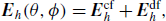(1)

where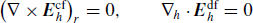(2)

and subscript h denotes the horizontal components. In Subsections 2.1 and 2.2 we will show that the divergence-free electric field is purely inductive and can be calculated from Faraday’s law, while the curl-free part is associated with charge accumulation caused by horizontal conductivity gradients in the ground.

It should be noted that the decomposition into curl- and divergence-free parts is unique only if it’s done globally. In regional studies where the analysis area is some hundreds or thousand km across, there may be an additional “Laplacian” part that has zero curl and zero divergence inside the analysis area. In this study we will neglect the Laplacian part, which may cause some errors in regional studies. However, the errors are expected to diminish as the analysis area increases.

The above division into curl- and divergence-free parts can be compared with the common practice in magnetotel-lurics, where the electromagnetic surface field is divided into tangential-electric (TE) and tangential-magnetic (TM) modes (e.g. Becken et al., 2008; Chave and Weidelt, 2012). In the TE (TM) mode the electric (magnetic) field has no vertical component at the ground surface. In the general case with 3D ground conductivity variations the TE and TM modes are coupled to each other, but in 2D cases they can be separated into E-polarization and B-polarization modes by choosing the coordinate system appropriately. With 1D ground conductivity structure (layered in vertical direction) the TE and TM modes degenerate to the same poloidal-magnetic mode. This is often assumed as the normal mode in MT studies, so that the anomalous fields are created by additional 2D or 3D conductivity structures.

The 1D normal mode is purely inductive, and so is the whole TE mode in a 2D situation. In contrast, the anomalous part of the 2D TM mode (i.e. deviation from the normal mode) is caused by charge accumulation at the conductivity gradient. In the general 3D situation the TE and TM modes are mixed, but it is still reasonable to assume that TE mode is mostly inductive while the anomalous part of TM mode is heavily affected by charge accumulation (Becken et al., 2008). Thus we see that E df h in Eq. (1) can be identified with the TE mode (here including the normal mode), while E cf h is associated with the anomalous part of the TM mode.

### 2.1 Divergence-free electric field

We can solve the divergence-free part of the electric field directly from Faraday’s law,(3)

using the measured vertical magnetic disturbance B r as the input data. We can represent the divergence-free electric field in terms of a potential, as, so that Eq. (3) forms a Poisson’s equation for ψ. However, there are two issues in solving Eq. (3) this way. We must interpolate the vertical magnetic field inside the (sometimes rather sparse) magnetometer network in a physically sensible way and unless we have global data coverage we have to specify some boundary conditions for ψ.

Becken and Pedersen (2003) and Becken et al. (2008) show how the TE mode impedance tensor and electric field can be calculated from measured tipper vectors, without knowing the 3D ground conductivity structure. In their method the hypothetical normal magnetic disturbance is given, and the anomalous TE mode magnetic field is solved iteratively. Then the anomalous TE mode surface electric field can be calculated by solving Faraday’s law in Eq. (3) in frequency/wavenumber domain. The total TE electric field, equal to the divergence-free field E df h , is obtained by adding the normal part from a separate (distant) measurement. While this approach seems to work well, and might be useful for hypothetical event analysis of GIC characteristics, we want to develop a more straightforward space/time domain algorithm, where previously measured tipper vectors are not required.

One convenient way to solve Eq. (3) in space/time domain is the Spherical Elementary Current Systems (SECS) method introduced by Amm (1997) and Amm and Viljanen (1999). In the SECS method the magnetic disturbance field is represented in terms of an equivalent current that is composed as a sum of elementary systems. It has been shown to be a very flexible and robust method for determining ionospheric equivalent currents (Amm and Viljanen, 1999; Weygand et al., 2011), separating the ground magnetic variation field into internal and external parts (Pulkkinen et al., 2003b) and interpolating the surface magnetic field (Pulkkinen et al., 2003a; McLay and Beggan, 2010).

The external equivalent current Jeq,ext is defined as a divergence-free spherical sheet current that gives the same magnetic field below the ionosphere as the real external (ionospheric + magnetospheric) currents. According to the potential theory such a solution always exists and is uniquely defined in global scale (see discussion in Haines and Torta, 1994; Amm and Viljanen, 1999). However, the measured magnetic disturbance also has internal (induced) sources, in addition to the external currents. This means that we cannot represent all 3 components of the disturbance field using Jeq,ext alone. Rather, we can represent either the vertical or the horizontal part with J,eq ext. In previous GIC studies emphasis has often been on the horizontal magnetic field (e.g. Viljanen et al., 2001; Pulkkinen et al., 2003a and references therein), but in this study the vertical magnetic field is needed in Faraday’s law.

We note that in principle we could model the whole measured magnetic disturbance field, both vertical and horizontal parts at the same time, by adding another layer of internal equivalent current to model the internal sources, as done e.g. by Pulkkinen et al. (2003b). We tested this two-layer approach with the numerical examples described in Section 3, but concluded that it does not significantly improve the solution for the surface electric field. Explanation is that according to potential theory the vertical part of the magnetic disturbance field can be represented solely by Jeq,ext, even if part of the disturbance is caused by internal sources (see discussions by Haines and Torta, 1994; Amm and Viljanen, 1999). Additional Jeq,int are needed only if both vertical and horizontal disturbances are modeled at the same time.

The divergence-free SECS functions defined by Amm (1997) and illustrated in Fig. 1 form a set of basis functions for representing any (continuously differentiable) divergence-free vector field on a sphere. The external equivalent current is determined so that elementary systems are placed in a suitable grid at a higher altitude and their magnitudes are chosen so that the resulting magnetic field fits the observations as closely as possible (e.g. in the least squares sense). Usually the equivalent current layer is placed at ~ 100 km, just below the real ionospheric currents, but smaller heights can be used to interpolate the magnetic measurements at dense magnetometer networks. In this study we use 100 km height in order to filter out smaller scale structures that are not really needed at GIC studies, although it means that agreement with local surface electric field measurements (see Subsection 3.3) may get slightly worse. More details about grid selection and the fitting process are given e.g. by Amm and Viljanen (1999), Pulkkinen et al. (2003b) and Weygand et al. (2011).

For each individual time-step we collect the vertical components of the measured ground magnetic disturbance at locations r n = (R e , θ n , ϕ n ) into one vector(4)

while the unknown scaling factors of the elementary systems located at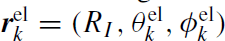are collected into another vector(5)

Here R e is Earth’s radius and R I > R e is the radius of the external (ionospheric) equivalent current layer. These vectors are connected by a transfer matrix T, so that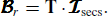(6)

Elements of the transfer matrix T give the vertical magnetic field caused by each individual unit SECS at the magnetometer sites, and is therefore known and depends only on geometry. For example, T n, k gives the vertical magnetic field at r n caused by the SECS centered at r el k . Details how to calculate the matrix T are given in Appendix A, while Amm and Viljanen (1999) and Weygand et al. (2011) discuss how to invert Eq. (6) for the unknown scaling factors secs using truncated singular value decomposition.

The inversion of Eq. (6) is done for each time-step separately, but using the same elementary system grid (locations r el k ). This means that the matrix T has to be inverted just once for each magnetometer network. In this study our elementary system grid is located between 56°−73° North and −8°−52° East, with 0.6° resolution in latitude and 1.4° in longitude, so that it extends few degrees outside the continental BEAR and IMAGE magnetometer networks shown in Fig. 2. The optimum truncation point in SVD for the IMAGE, BEAR and “Ideal” networks used in Section 3 was chosen to minimize the least-squares error in the resulting electric field. Thus 13 out of 18, 16 out of 36 and 154 out of 825 singular values were used in analyzing the data from IMAGE, BEAR and “Ideal” networks, respectively.

Once the scaling factors secs of the elementary systems are determined from Eq. (6), we can calculate the vector potential A associated with the external equivalent current. We need only the horizontal components of the vector potential at the Earth’s surface, at locations (r m = (R E , θ m , φ m ). It can be calculated as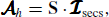(7)

where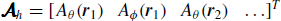(8)

contains the horizontal components of the vector potential and S is another transfer matrix that is described in Appendix B. According to the definition of the vector potential B = × A and in the Coulomb gauge used by Amm and Viljanen (1999) h h = 0, so we can calculate the divergence-free part of the surface electric field from Eq. (3) simply as(9)

### 2.2 Curl-free electric field

The curl-free part of the surface electric field is more difficult to calculate. As discussed above and in Becken et al. (2008), it is equal to the anomalous part of TM mode electric field, and vanishes if there are no horizontal gradients in ground conductivity (1D layered ground).

If the conductivity σ(RE, θ, ϕ) of the ground surface layer is known, we can write the surface current as(10)

The total current is divergence-free, so divergence of the surface current can be written as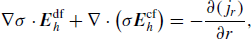(11)

where −j r is the vertical current flowing downwards from the surface. If we could assume that vertical currents near the ground surface are vanishingly small, we could solve the curl-free part of the surface electric field from Eq. (11), assuming that the surface conductivity is known. Another option is to use the anomalous part of the TM mode impedance tensor, which can be deduced from the total and TE mode impedance tensors as shown by Becken et al. (2008). However, if the total impedance tensor were known in the whole analysis area with good enough spatial resolution, then the surface electric field could be calculated directly from the measured (and interpolated) magnetic field, without any need to solve Eqs. (3) and (11). This is rarely the situation in GIC studies, although McKay and Whaler (2006) have used this approach in a rather small spatial area.

Equation (11) shows how divergence of E cf h is associated with sharp conductivity gradients, such as coast lines (e.g. Jiracek, 1990; Gilbert, 2005), where the term Δσ is large. However, the electric field E cf h itself may extend hundreds or even thousand km outside these regions (e.g. Ferguson et al., 1990).

In the present study we will simply assume that curl-free part of the electric field is zero,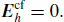(12)

This is equivalent to ignoring the TM mode. The main reason for this assumption is that our primary goal in this study is to find a simple way to estimate the surface electric field directly from magnetic data, without any knowledge of the ground conductivity. An attempt to solve E cf h from Eq. (11) would violate this principle. However, we also note that in present GIC studies it is common practice to use calculation methods where the curl-free electric field is ignored, e.g. plane wave method combined with 1D ground conductivity structure (Viljanen et al., 2004), and these seem to give useful results. This indicates that in many (or even most) situations the divergence-free electric field is much more important than the curl-free field, at least in the spatial scales involved in GIC modeling.

However, it should be kept in mind that our assumption in Eq. (12) is a reasonable approximation at best, and in some situations, where charge accumulation due to large conductivity gradients dominates the surface electric field, it may be completely unrealistic. Thus our calculation method described in Subsection 2.1 produces only the divergence-free part of the electric field, equivalent to the sum of the TE-mode and non-anomalous part of the TM-mode. This approximation may well explain why some of our results with BEAR data (discussed in Subsection 3.3) were less than optimal.

## 3. Test Examples

We can test the calculation method presented in the previous section by constructing realistic test cases where the correct surface electric field is known. We also present one test case using magnetic and electric measurements from the Baltic Electromagnetic Array Research (BEAR) network, which operated for about 1.5 months during June-July 1998 (Korja et al., 1998).

### 3.1 Electrojet

Our first test case is a simplified oscillating electrojet, which we model by placing a line current at an altitude H above the Earth’s surface. According to Boteler et al. (2000) this is equivalent to a sheet current at a lower altitude with a Cauchy-type current distribution. Ground induction is modeled by placing a perfect conductor at depth h below the surface. We place the electrojet at latitude 65°, directly above the IMAGE and BEAR networks illustrated Fig. 2.

For simplicity we calculate the magnetic and electric fields caused by the oscillating electrojet in Cartesian geometry (ê x North, ê y East, ê z down) by making a stereographic projection for the station coordinates. For an East-West oriented line current the surface fields are simply (Boteler et al., 2000)(13)(14)

where z = 0 is the ground surface and the line current is centered at x = 0. In our example we use values H = 300 km, h = 50 km and I = 106 sin(ω t) with ω = 2π/300 s−1. These fields are evaluated at the IMAGE and BEAR stations and the radial magnetic field is used as input in the SECS-based calculation method, without correcting for the difference between local Cartesian and spherical geometry. The IMAGE and BEAR networks are relatively small (we neglect stations above 72° North, see Fig. 2), so this simplification does not cause significant errors.

The correct North-South profiles of the surface electric and magnetic fields as well as the results calculated using t B r from the IMAGE and BEAR stations are illustrated in Fig. 3, which shows a cut along 23° meridian. From the upper panel we see that the vertical part (z-component) of the magnetic field is very well reproduced from the BEAR observations, and even the sparser IMAGE array gives a reasonable estimate. In contrast, the middle panel shows that the horizontal part (in this case x-component) is coarsely underestimated. This is a direct consequence of the calculation process where only the vertical field is used as the input, as the ground induced currents tend to decrease the vertical magnetic field but increase the horizontal field. Results for the horizontal surface electric field (in this case y-component) are illustrated in the bottom panel. Taking into account the small distortions caused by the simplified geometry, we conclude that the electric field calculated from the BEAR network is in very good agreement with the model and even the more sparse IMAGE array gives reasonable results for the 1D electrojet.

### 3.2 Data-based model

A more concrete test can be performed by using realistic, data-based models of typical ionospheric current systems. Here we use model of a westward traveling surge (WTS) constructed by Amm (1995). Ground induction is modeled using the Complex Image Method (CIM, Wait and Spies, 1969; Thomson and Weaver, 1975; Boteler and Pirjola, 1998; Pirjola and Viljanen, 1998). The CIM calculation gives the electric and magnetic fields at the Earth’s surface as a sum of external and internal parts, once the temporal evolution of the primary external (ionospheric) current system and 1D Earth conductivity structure are specified. In this study we use a layered ground model that is suitable for Central Finland (see figure 4 by Viljanen and Pirjola, 1994) and create time evolution of the WTS system by moving the static model westward at a constant speed of 10 km/s. More discussion about the WTS model as well as the CIM calculation process are given by Vanhamäki at al. (2005), who used the same approach to study the feedback effect of ground induction on the ionospheric current system.

Figure 4 shows the surface electric field calculated for the WTS model using the CIM procedure (top left panel), as well as the electric fields estimated from B r using simulated measurement at different magnetometer networks. The result labeled as “Ideal” makes use of B r aat every available point of the CIM modeling area, which is a 1100 km (latitude) × 1800 km (longitude) grid with 50 km spacing in both horizontal directions. The result from this Ideal network is in excellent agreement with the original model. Also the result based on the simulated measurements at the BEAR stations (bottom left panel) captures the overall structure of the surface electric field and also the magnitude is reasonably accurate. The main error in the BEAR result seems to be underestimation of the electric field magnitude and spreading of small-scale features into too large areas.

On the other hand, the result based on the IMAGE network is practically useless. This may be caused by the fact that according to Fig. 4 the scale size of the WTS system is smaller than the typical magnetometer separation of the IMAGE array. However, the moderate deterioration of our results when changing from the Ideal network to the dense BEAR, compared to the large drop in quality when changing to the more sparse IMAGE may also indicate some intrinsic limitation of the calculation method with respect to spatial resolution of the input data.

It should be noted that in the previous electrojet example and in this WTS test the surface electric field is purely rotational. The curl-free part of the surface electric field is exactly zero, as there are no horizontal gradients in ground conductivity. Thus our calculation method may have given too promising results, as in reality our assumption in Eq. (12) is never exactly satisfied.

### 3.3 BEAR data

Our final test utilizes direct measurements of the surface electric and magnetic fields collected with the BEAR network. The largest event during BEAR operation, a sub-storm with maximum local AE index of ~1300 nT, took place in the morning hours of 26 June 1998. The surface electric field recorded at most of the BEAR stations was usually severely modified by local conductivity anomalies, but there are a few stations where the measured E h should give a good estimate of the large scale surface electric field around the station (T. Korja, private communication).

We use the electric field measured during the substorm on 26 June 1998 at stations Jokkmokk and Boden (see Fig. 4) as our test cases. We use the vertical magnetic variations observed at all other BEAR stations (i.e. excluding magnetic data from the station being studied) as the input data and calculate the surface electric field at Jokkmokk and Boden using the SECS technique. Figures 5 and 6 show the measured electric fields and the results of our calculation. For clarity we have plotted 1-minute averages taken from 10-second data. Agreement between the measurement and calculation is very good, except for the x-component at Jokkmokk. The correlation coefficients are −0.22 and 0.82 for x- and y-components at Jokkmokk, and 0.72 and 0.64 for the x- and y-components at Boden, respectively.

We should point out that these examples, with the exception of x-component at Jokkmokk, are the best result we were able to obtain for the BEAR data. For other stations our results were less impressive, although correlations larger than 0.6 were obtained also on some other nearby stations. It can be speculated that worse results on other stations, as well as the poor result for the x-component at Jokkmokk, could be explained in terms of local conductivity effects or galvanic disturbances on the electric field that are known to be a problem at many BEAR stations (T. Ko-rja, private communication). Also the limited area of magnetic data availability is expected to affect our calculation results near the edges of the BEAR network. However, another possible limiting factor is the fact that also the vertical magnetic field is rather sensitive to local conductivity anomalies (as opposed to the more commonly used horizontal magnetic field), which would mean that very dense magnetometer network are required. And we must of course keep in mind that we completely neglect the curl-free part of the electric field. At the moment we cannot estimate how significantcould be in the Fennoscandian area, but it’s known that in some situations it may affect the surface electric field at considerable distances around conductivity gradients (e.g. Ferguson et al., 1990). A more thorough study using detailed magnetotelluric data available from the area (e.g. Korja et al., 2002; Lahti et al., 2005) would be required, before these issues can be reliably identified and resolved.

## 4. Summary and Discussion

We have demonstrated that it is possible to estimate the geomagnetically induced electric field at the Earth’s surface directly from the time derivative of the vertical magnetic field, without any knowledge of the underlying ground’s electric conductivity. The basic idea is to i) separate the surface electric field into curl-free and divergence-free parts, ii) solve the divergence-free part from Eq. (3) and iii) neglect the curl-free part. Step i) can always be done, step ii) is theoretically exact if we only had perfect knowledge of B r aat the ground surface and step iii) is valid if we neglect horizontal conductivity gradients in the Earth. In regions where conductivity gradients are important, our result is approximative and amounts to neglecting the anomalous part of the TM-mode.

Our approach is based on methods developed by Vanhamäki et al. (2007) and Vanhamäki (2011) for ionospheric induction studies. Similar integration of Faraday’s law has been previously used by Becken and Pedersen (2003) and Becken et al. (2008) in MT studies. However, their primary interest is in the impedance tensor ground conductivity structure, while the surface electric field is a side-product. Moreover, as is usual practice in MT studies, their method works in the frequency/wavenumber domain and assumes quasi-uniform sources. Becken et al. (2008) show that the TE part of the electric field (corresponding to our Edf) can be calculated using only magnetic tipper vectors as the input data, without knowing the detailed ground conductivity structure or the whole surface impedance tensor. While this method might be useful in hypothetical event analysis, e.g. estimating possible GIC levels in a given network, in many GIC applications a time/space domain approach that makes no assumptions about ionospheric sources is more appropriate.

Our new solution algorithm for the divergence-free part of the surface electric field is based on the use of external equivalent current, which offers a physics-based way to represent the magnetic variations and associated electric field. It should be noted that Jeq,ext are enough to represent the whole vertical magnetic disturbance B r , even if part of the disturbance is created by internal induced currents. The Spherical Elementary Current Systems method is a convenient way to calculate the equivalent current, as it can be easily adapted to accommodate the the spatial extent and shape of various magnetometer networks. Additionally, the individual elementary systems are simple enough to allow analytical calculation of the electric and magnetic fields.

We note that in the SECS analysis we don’t have to provide any explicit boundary conditions for the surface electric field. However, the implicit and automatically included boundary condition is that the equivalent current does not have any sources outside the analysis area ( × Jeq,ext = 0 outside), which means that we neglect the possible Lapla-cian part of the electric field in Eq. (1). This is usually an incorrect assumption, and distant current systems are expected to affect the observed Eh, especially near the boundaries of the analysis area. However, boundary effects can be reduced by extending the elementary system grid some distance outside the area of interest.

In the present analysis we assume that the curl-free part of the surface electric field is zero, which is equivalent to ignoring the magnetotelluric TM mode. This seems to be a reasonable assumption in many situations, as even the simplified plane wave model with a layered Earth model is able to reproduce GIC with good accuracy (Viljanen et al., 2004). However, in general we expect that the surface electric field may have a significant curl-free part near sharp conductivity gradients, such as ocean-land interfaces. In principle we could attempt to estimate the curl-free part from Eq. (11) or by using previously measured impedance tensor, but that would violate our main goal in this article, which is estimating the electric field using only directly measured magnetic data.

We have tested the new calculation method with an idealized electrojet, realistic data-based models as well as actual data from the BEAR network. Results for the WTS model indicates that if we had data from an ideal magnetometer network, the new SECS-based calculation method would give an almost exact result for the surface electric field. Also the results for the temporary BEAR network are quite accurate. Analysis of the substorm event on 26 June 1998 proves that with magnetic data from the BEAR stations we can calculate the electric field at reference stations. However, a more detailed study is needed to determine how large contribution the presently ignored curl-free electric field (magnetotelluric TM mode) makes to the total large-scale surface electric field in the Fennoscandian region, and how it would affect GIC modeling.

In the electrojet test case results based on the IMAGE data were reasonably accurate. However, in many situations the permanent IMAGE network (at the present configuration) may be too sparse for the new SECS-based calculation method, as the IMAGE-based results for the smaller scale WTS model were practically useless. This is a disadvantage compared to several previous methods for estimating the surface electric field, as there are methods that work quite well with IMAGE data (e.g. Viljanen et al., 2004). The main theoretical advantage of the presented method is that there is no need to know the Earth conductivity distribution. However, it would appear that in practice it isn’t too hard to obtain a conductivity model that is accurate enough for GIC purposes (Thomson et al., 2009).

We conclude that the principle of calculating the divergence-free part of the surface electric field from time derivative of the vertical magnetic field is sound, and the method works also in practice. However, at present time practical applications may be somewhat limited as the method seems to require data from a relatively dense and spatially extended magnetometer network. However, a more thorough study is needed to evaluate the practical applicability of the presented method in various situations, taking into account e.g. the spatial extent and distribution of the magnetometer array as well as the role of the curl-free electric field caused by charge accumulation at horizontal conductivity gradients.

## References

• Amm, O., Direct determination of the local ionospheric Hall conductance distribution from two-dimensional electric and magnetic field data: Application of the method using models of typical ionospheric electrody-namic situations, J. Geophys. Res., 100, 21473–21488, 1995.

• Amm, O., Ionospheric elementary current systems in spherical coordinates and their application, J. Geomag. Geoelectr., 49, 947–955, 1997.

• Amm, O. and A. Viljanen, Ionospheric disturbance magnetic field continuation from the ground to the ionosphere using spherical elementary current systems, Earth Planets Space, 51, 431–440, 1999.

• Becken, M. and L. B. Pedersen, Transformation of VLF anomaly maps into apparent resistivity and phase, Geophysics, 68, 497–505, 2003.

• Becken, M., O. Ritter, and H. Burkhardt, Mode separation of magnetotel-luric responses in three-dimensional environments, Geophys. J. Int., 172, 67–86, 2008.

• Boteler, D. H. and R. Pirjola, The complex-image method for calculating the magnetic and electric fields produced at the surface of the Earth by the auroral electrojet, Geophys. J. Int., 132, 31–40, 1998.

• Boteler, D. H., R. Pirjola, and L. Trichtchenko, On calculating the electric and magnetic fields produced in technological systems at the Earth’s surface by a “wide” electrojet, J. Atmos. Sol.-Terr. Phys., 62, 1311–1315, 2000.

• Chave, A. D. and P. Weidelt, The theoretical basis for electromagnetic induction, in The Magnetotelluric Method, edited by A. D Chave and A. G. Jones, 552 pp., ISBN 978-0-521-81927-5, Cambidge University Press, Cambridge, 2012.

• Egbert, G. D., Processing and interpretation of electromagnetic induction array data, Surv. Geophys., 23, 207–249, 2002.

• Ferguson, I. J., F E. M Lilley, and J. H. Filloux, Geomagnetic induction in the Tasman Sea and electrical conductivity structure beneath the Tasman Seafloor, Geophys. J. Int., 102, 299–312, 1990.

• Gilbert, J. L., Modeling the effect of the ocean-land interface on induced electric fields during geomagnetic storms, Space Weather, 3, S04A03, doi:10.1029/2004SW000120, 2005.

• Gough, D. I., Magnetometer array studies, Earth structure and tectonic processes, Rev. Geophys., 27, 141–157, 1989.

• Groom, R. W. and K. Bahr, Corrections for near surface effects: Decomposition of the magnetotelluric impedance tensor and scaling corrections for regional resistivies: A tutorial, Surv. Geophys., 13, 341–379, 1992.

• Haines, G. V. and J. M. Torta, Determination of equivalent current sources from spherical cap harmonic models of geomagnetic field variations, Geophys. J. Int., 118, 499–514, 1994.

• Jiracek, G. R., Near-surface and topographic distortions in electromagnetic induction, Surv. Geophys., 11, 163–203, 1990.

• Jozwiak, W., Large-scale crustal conductivity pattern in central Europe and its correlation to deep tectonic structures, Pure Appl. Geophys., doi:10.1007/s00024-011-0435-7, 2011.

• Korja, T. and the BEAR Working Group, BEAR: Baltic Electromagnetic Array Research, EUROPROBE News, 12, 4–5, 1998.

• Korja, T., M. Engels, A. A. Zhamaletdinov et al., Crustal conductivity in Fennoscandia—A compilation of a database on crustal conductance in the Fennoscandian Shield, Earth Planets Space, 54, 535–558, 2002.

• Lahti, I., T. Korja, P. Kaikkonen, K. Vaittinen, and BEAR Working Group, Decomposition analysis of the BEAR magnetotelluric data: implications for the upper mantle conductivity in the Fennoscandian Shield, Geophys. J. Int., 163, 900–914, 2005.

• McKay, A. J. and K. A. Whaler, The electric field in northern England and southern Scotland: Implications for geomagnetically induced currents, Geophys. J. Int., 167, 613–625, doi:10.1111/j.1365-246X.2006.03128.x, 2006.

• McLay, S. A. and C. D. Beggan, Interpolation of externally-caused magnetic fields over large sparse arrays using Spherical Elementary Current Systems, Ann. Geophys., 28, 1795–1805, doi:10.5194/angeo-28-1795-2010, 2010.

• Pirjola, R., Geomagnetic effects on ground-based technological systems, in Review of Radio Science 1999–2000, edited by W. R. Stone, 24 pp., ISBN 978-0-471-26866-6, Wiley-IEEE Press, New York, 2002.

• Pirjola, R. and A. Viljanen, Complex image method for calculating electric and magnetic fields produced by an auroral electrojet of finite length, Ann. Geophys., 16, 1434–1444, 1998.

• Pulkkinen, A., O. Amm, A. Viljanen, and BEAR Working Group, Ionospheric equivalent current distributions determined with the method of spherical elementary current systems, J. Geophys. Res., 108, 1053, doi:10.1029/2001JA005085, 2003a.

• Pulkkinen, A., O. Amm, A. Viljanen, and BEAR Working Group, Separation of the geomagnetic variation field on the ground into external and internal parts using the spherical elementary current system method, Earth Planets Space, 55, 117–129, 2003b.

• Pulkkinen, A., A Thomson, E. Clarke, and A. McKay, April 2000 geomagnetic storm: Ionospheric drivers of large geomagnetically induced currents, Ann. Geophys., 21, 709-717, 2003c.

• Thomson, A. W. P, A. J. McKay, and A. Viljanen, A review of progress in modelling of induced geoelectric and geomagnetic fields with special regard to induced currents, Acta Geophys., 57, 209-219, doi:10.2478/s11600-008-0061-7, 2009.

• Thomson, D. and J. Weaver, The complex image approximation for induction in a multilayered Earth, J. Geophys. Res., 80, 123–129, 1975.

• Vanhamäki, H., Inductive ionospheric solver for magnetospheric MHD simulations, Ann. Geophys., 29, 97–108, 2011.

• Vanhamäki, H., A. Viljanen, and O. Amm, Induction effects on ionospheric electric and magnetic fields, Ann. Geophys., 23, 1735–1746, 2005.

• Vanhamäki, H., O. Amm, and A. Viljanen, Role of inductive electric fields and currents in dynamical ionospheric situations, Ann. Geophys., 25, 437–455, 2007.

• Viljanen, A. and R. Pirjola, On the possibility of performing studies on the geoelectric field and ionospheric currents using induction in power systems, J. Atmos. Terr. Phys., 56, 1483–1491, 1994.

• Viljanen, A., H. Nevanlinna, K. Pajunpää, and A. Pulkkinen, Time derivative of the horizontal geomagnetic field as an activity indicator, Ann. Geophys., 19, 1107–1118, 2001.

• Viljanen, A., A. Pulkkinen, O. Amm, R. Pirjola, T. Korja, and BEAR Working Group, Fast computation of the geoelectric field using the method of elementary current systems and planar Earth models, Ann. Geophys., 22, 103–113, 2004.

• Wait, J. R. and K. P. Spies, On the image representation of the quasi-static fields of a line current source above the ground, Can. J. Phys., 47,2731–2733, 1969.

• Weygand, J. M., O. Amm, A. Viljanen, V. Angelopoulos, D. Murr, M. J. Engebretson, H. Gleisner, and I. Mann, Application and validation of the spherical elementary currents systems technique for deriving ionospheric equivalent currents with the North American and Greenland ground magnetometer arrays, J. Geophys. Res., 116, A03305, doi:10.1029/2010JA016177, 2011.

• Zhang, P., L. B. Pedersen, M. Mareschal, and M. Chouteau, Channelling contribution to tipper vectors: A magnetic equivalent to electrical distortion, Geophys. J. Int., 113, 693–700, 1993.

## Acknowledgments

The work of H. Vanhamäki was supported by the Academy of Finland (project number 126552). We thank all institutes maintaining the IMAGE magnetometer network (http://space.fmi.fi/image/). The BEAR project was partly supported by INTAS (no. 97-1162).

## Author information

Authors

### Corresponding author

Correspondence to Heikki Vanhamäki.

## Appendices

### Appendix A.

According to Eq. (9) of Amm and Viljanen (1999), the radial magnetic field of a divergence-free SECS below the current layer (ionosphere) is given by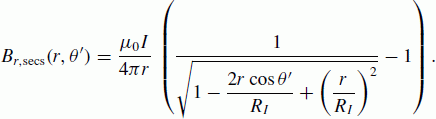(A.1)

Here I is the scaling factor of the elementary system (to be solved from Eq. (6)) and θ′ is the co-latitude of the calculation point expressed in a coordinate system that is centered at the SECS pole. See Fig. A.1 for reference.

The components of the matrix T in Eq. (6) are given simply by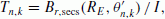(A.2)

whereis the angle between calculation point r n = (R E , θ n , φ n and SECS location. According to spherical trigonometry it can be expressed as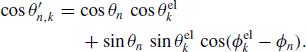(A.3)

### Appendix B.

According to equation (A.6) of Amm and Viljanen (1999), the vector potential of a divergence-free SECS below the current layer (ionosphere) is given by(B.1)

Here the unit vector êφ, is given in the coordinate system centered at the SECS pole. From Fig. A.1 we can see that in the geographical coordinate system the unit vector can be expressed as(B.2)

It is a straightforward exercise in spherical trigonometry to show that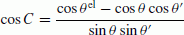(B.3)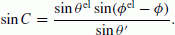(B.4)

Using Eqs. (A.3–B.4) we can evaluate components of the matrix S from expressions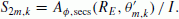(B.5)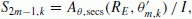(B.6)

## Rights and permissions

The images or other third party material in this article are included in the article’s Creative Commons licence, unless indicated otherwise in a credit line to the material. If material is not included in the article’s Creative Commons licence and your intended use is not permitted by statutory regulation or exceeds the permitted use, you will need to obtain permission directly from the copyright holder.

To view a copy of this licence, visit https://creativecommons.org/licenses/by/4.0/.

Reprints and Permissions

Vanhamäki, H., Viljanen, A., Pirjola, R. et al. Deriving the geomagnetically induced electric field at the Earth’s surface from the time derivative of the vertical magnetic field. Earth Planet Sp 65, 997–1006 (2013). https://doi.org/10.5047/eps.2013.03.013

• Revised:

• Accepted:

• Published:

• Issue Date:

• DOI: https://doi.org/10.5047/eps.2013.03.013

### Key words